Mobile QR Code1. (Samsung Electronics Company, Limited, Hwaseong 18448, Korea)
2. (Samsung Display Company, Limited, Yong-in 17113, Korea)
3. (Department of Electronic Engineering, Sogang University, Seoul 04107, Korea)

Active-matrix organic light emitting diodes (AMOLED), column driver integrated circuit (IC), digital-to-analog converter (DAC), ramp signal, two-step interpolation

## I. INTRODUCTION

Recently, high-resolution displays such as wide quad extended graphics array (WQXGA) and ultra-high definition (UHD) panels have been widely employed in mobile devices such as smart phones and tablet PC’s. Demand for active-matrix organic light emitting diodes (AMOLED) to maintain low power consumption at high-resolution has also increased. With the rapidly increasing demand for high-resolution panels including AMOLED panels, there have been a growing number of studies conducted on the development of improved multi-channel column driver integrated circuits (IC's). For those column driver IC’s, an 8- to 10-bit digital-to-analog converter (DAC) is required to convert digital image signals into analog signals. In the conventional column driver IC’s, a resistor DAC (RDAC) based on global resistor strings, typically demonstrating dependable output uniformity among channels, has been commonly used [1-3]. However, in the case of RDAC’s, it has been difficult to efficiently reduce the area of the column driver IC, considering that $2^n$ reference voltages and $2^{n+1-2}$ analog switches are required. Two-step interpolation structures, where the RDAC is employed in the first stage, have been previously developed [4-10] and have been found to both increase area efficiency and maintain the channel uniformity among channels. However, since the first-stage RDAC commonly should process more than 6 bits in order to maintain uniformity among the channels, it is not easy to reduce the area.

As an example, an UHD display system with a frame rate of 60 Hz is shown in Fig. 1, where six 960-channel column driver IC’s are used with the 2:1 DEMUX function. In general, since a single DAC is used per unit channel in the column driver IC, it is difficult to reduce the chip area of the column driver IC in the high-resolution display panel.

In order to increase the area efficiency of the RDAC-based column driver IC, a time-shared DAC architecture in which a single DAC is shared among multiple channels was proposed in . However, since the single DAC is shared among multiple channels during the limited 1-horizontal (1-H) time, a high operation speed is needed in the time-shared DAC structure. In addition, to improve the settling behavior of the global reference voltages within the wide width of the column driver IC, a large amount of power consumption is required. Since the 1-H time is also shared among the shared DAC and the output amplifiers, it is not easy to optimize the power consumption of the column driver IC.

The effective 1-H time of the column driver IC used in 60 Hz/frame UHD AMOLED panels, which are currently in high demand, is approximately 3.5 μs. Compared to the 15.4 μs of the 60 Hz/frame full high definition (FHD) panels, the operation speed of the aforementioned AMOLED panels is approximately four times faster.

This paper proposes a ramp signal-based column driving technique, which is able to reduce the area of the column driver IC of 60 Hz/frame UHD AMOLED panels. Using the single-line ramp signal, which variesconsecutivelyin time, each channel is able to sample and hold an analog voltage corresponding to the digital input. At this time, to reduce the required operation speed of the ramp signal within the limited 1-H time, a two-step interpolation structure composed of a first-stage 6-bit ramp signal and a second-stage 2-bit amplifier DAC (amp DAC) is employed.

Fig. 2. Conventional $k$-Channel Column Driver IC Based on the Channel RDAC Structure.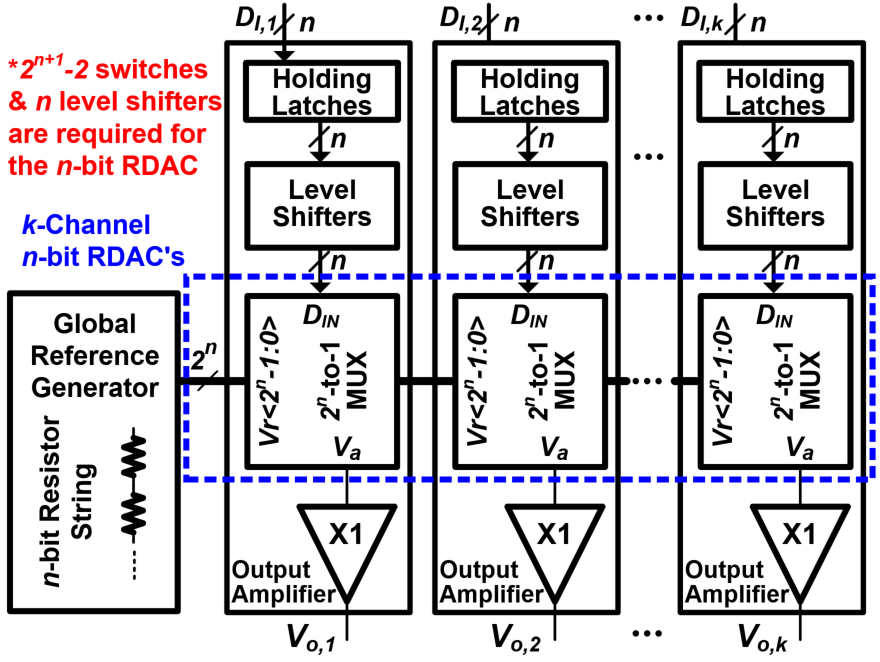This paper is organized as follows. Section Ⅱ details the proposed ramp signal-based column driving technique and its operating principle. Section Ⅲ summarizes the proposed circuit design techniques. The fabricated and measured results of the 96-channel test chip are summarized in Section Ⅳ and the conclusion is given in Section Ⅴ.

## II. ARCHITECTURE

### 1. Concept of the Proposed Ramp Signal-based Column Driving Technique

The conventional column driver IC’s in AMOLED panels commonly use a channel RDAC structure based on global reference voltages as shown in Fig. 2. As the resolution increases, the number of the global reference voltage lines and channel analog switches also increases exponentially.

Two-step interpolation structures have been employed commonly to reduce the area of the high-resolution RDAC, where the first-stage RDAC processes at least 6 bits to obtain uniformity among the channels. In AMOLED panels, since each RGB pixel is distinct from all others, the output characteristics of each global reference voltage have to be controlled individually. As a result, $3×2^6$global reference voltage lines are needed in the column driver IC for the AMOLED panel . In addition, $n$ level shifters of each channel, which occupy a large area, limit the realization of the small-area column driver IC.

Fig. 3. Proposed $k$-Channel Ramp Signal-Based Column Driver IC.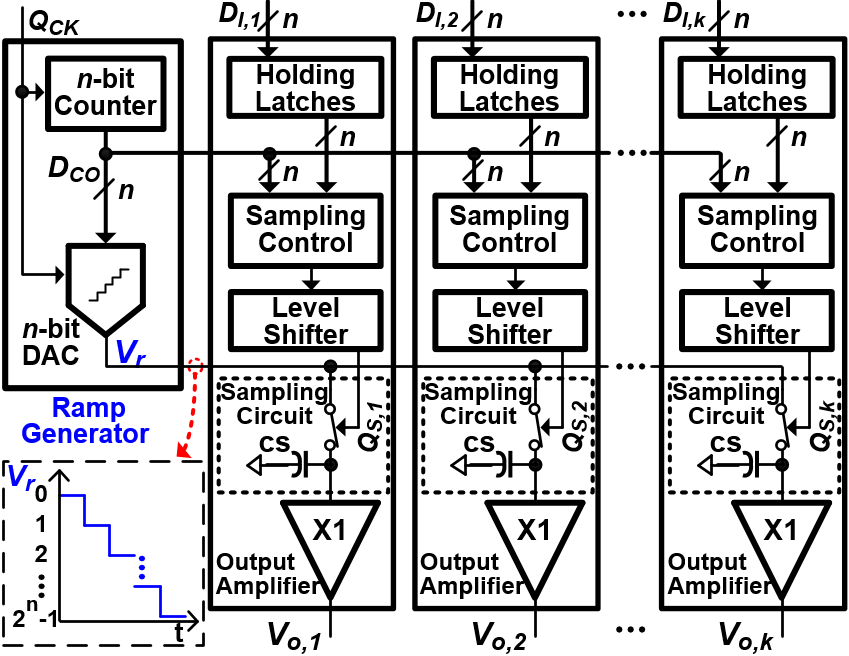In this paper,a ramp signal-based column driving technique is proposedto reduce the area of the column driver IC which increases exponentially with the resolution. The $k$-Channel column driver ICis described in Fig. 3, where the proposed column driving technique is applied. The ramp signal-based column driver IC employs only a single analog ramp signal and n-bit binary count signals which are synchronized with the main clock ($Q_{CK}$) and which change consecutively to all channels simultaneously. As a result, the area of the column driver IC is 44.3% reduced. Moreover, by reducing the number of level shifters for each channel from $n$ to 1, the area can be further reduced.

The proposed $k$-Channel ramp signal-based column driver IC is composed of an n-bit binary counter, a ramp signal generator, and k unit channel circuits. For the RGB-independent gamma characteristic of the AMOLED panel, three ramp signal generators should be used. Each unit channel circuit consists of holding latches, a sampling control circuit, a level shifter, a sampling capacitor, and an output amplifier. In the proposed column driver IC, the $2^n$ corresponding analog voltages are expressed as a ramp signal, changing consecutively, while delivered to all channels. Simultaneously, each channel uses the digital circuit composed of low-voltage devices to hold the analog voltage corresponding to the timing, reducing switching power consumption and active die area compared to the conventional column driver IC using high-voltage MUX’s.

Table 1. Operation Timing of the Proposed Ramp Signal-Based Column Driving Technique with an Example of 6-bit Operation.

 RDAC-based Column Driver IC Ramp-based Column Driver IC Holding Latches 26 μm 26 μm Level Shifters 60 μm 10μm Sampling Control Not Used 47 μm $2^n$-to-1 MUX (RGB) 384 μm Not Used Ramp Lines (RGB) Not Used 20 μm Sampling Circuit Not Used 90 μm Output Amplifier 155 μm 155 μm Total 625 μm 348 μm

Fig. 4. Operation Timing of the Proposed Ramp Signal-Based Column Driving Technique with an Example of 6-bit Operation.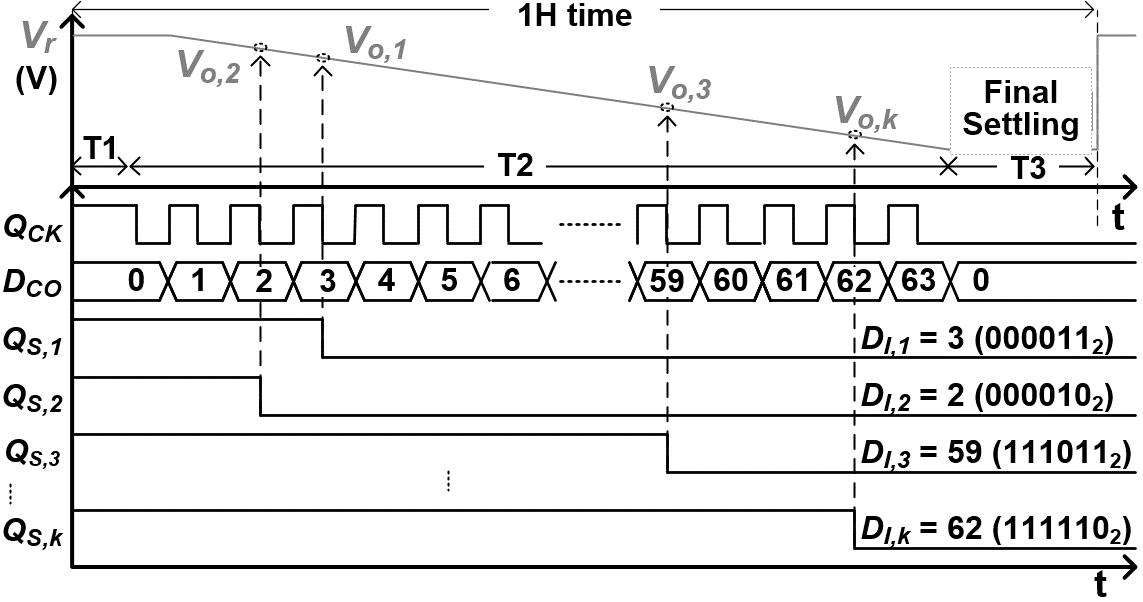The channel height comparison of the channel RDAC-based driver IC and the ramp signal-based driver IC is summarized in Table 1. For a fair comparison, a two-step interpolation architecture, which uses a 6-bit RDAC at the first stage, is employed in both column driver IC’s. The proposed ramp signal-based column driver IC shows a 44.3% area reduction effect, and it can maximize the area efficiency as the resolution of the driver IC increases.

### 2. Timing of the Proposed Ramp Signal-based Column Driving Technique

The 6-bit operation and timing examples of the proposed ramp signal-based column driving technique are described in Fig. 4 in detail. During the T1 period, the ramp signal ($V_r$) and the binary count signals ($D_{CO}$) are all reset. Then, $V_r$ and $D_{CO}$ increase by 1 LSB consecutively synchronized with $Q_{CK}$ and are delivered to all channels during the T2 period. The sampling control circuit of each channel compares two digital inputs, $D_I$ and $D_{CO}$, and changes the sampling signal ($Q_S$) from ‘high’ to ‘low’ when the two digital codes are identical. The sampling capacitor at the input node of the output amplifier tracks the ramp signal from the start of the T2 period. When $Q_S$ becomes ‘low’, the sampling switch turns off and the sampling capacitor holds the corresponding analog voltage.

However, in the proposed column driving architecture, the ramp signal has $2^n$ operation periods to represent n-bit image signals in the limited 1-H time and should be delivered to all channels within an operation period with the required settling accuracy. In the following Section Ⅲ, the design techniques to overcome the limitations are discussed.

### III. CIRCUIT IMPLEMENTATION

#### 1. Single-channel Architecture with Two-step Inter-polation

The proposed column driving technique employs a single ramp signal and n–bit binary count signal lines across all channels. Although the area of the column driver IC can be significantly reduced, $2^n$ operation periods depending on the resolution are still required for the ramp signal. Thus, to express 8-bit signals with 256 gray scales, the ramp signal must be changed consecutively for a total of 256 periods. For example, if the 1-H time is 3.5 μs, the ramp signal should be, taking into account the settlement margin of the output amplifier (= 1.0 μs), settled within approximately 10 ns with the required accuracy. In the case of a wide multi-channel column driver IC, a large amount of power is required to settle the ramp signal before it can be delivered to all channels within the required period of time which may be as short as 10 ns. Considering these requirementsin the proposed column driving technique, the proper two-step interpolation structure needs to be employed to reduce the operation periods of the ramp signal.

In the conventional two-step interpolation structures, two adjacent voltages to the first-stage DAC, Vh and Vl, are selected and the differential voltage between the two voltages is divided in the second stage. The three most common two-step interpolation structures are shown in Fig. 5(a). The resistor interpolation can lead to channel mismatch due to errors in the current supplied to the second resistor string [4-6]. The capacitor interpolation may result in nonlinear errors due to parasitic capacitance ($C_P$) [7,8]. The amplifier interpolation, which separates the input node of the output amplifier, is most commonly used in commercial products and has good linearity and channel uniformity [9,10]. Therefore, this work proposes an interpolation structure which combines amplifier interpolation and sampling timing control of the ramp signal as shown in Fig. 5(b).

Fig. 5. Two-Step Interpolation for a Single Channel (a) Conventional, (b) Proposed Interpolation Schemes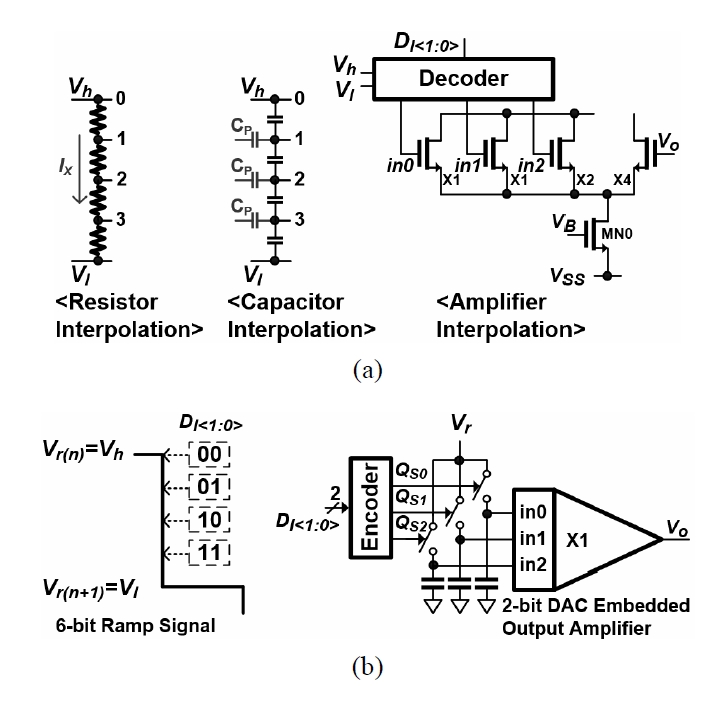The unit channel architecture of the proposed ramp signal-based column driver IC is described in Fig. 6. In this work, an 8-bit image signal is processed by the 6-bit ramp signal and the 2-bit amp DAC. The timing control circuit is implemented with simple digital logic circuits operating at a low supply voltage. The unit channel circuit receives the 6-bit digital count signal ($D_{CO}$), the ramp signal ($V_r$), the 8-bit digital input ($D_I$), and the main clock signal ($Q_{CK}$). The holding latches store $D_I$ during the 1-H period. The 6-bit digital comparator compares $D_{CO}$with 6 MSB’s of $D_I$ and decides its output of $Q_H$. When two digital codes are identical, $Q_H$ changes from ‘high’ to ‘low’. The 2-bit interpolation control circuit receives $Q_H$ and 2 LSB’s of $D_I$, and then generates three sampling signals, $Q_{S0}$, $Q_{S1}$, and $Q_{S2}$, to control the three separated sampling capacitors and sampling switches in the input node of the output amplifier.

The timing of the sampling and interpolation signals ($Q_{S1~3}$), depending on 2 LSB’sof $D_I$, is desribed in the right top table of Fig. 6. Each sampling capacitor samples $V_{r(n)}$ or $V_{r(n+1)}$ depending on 2 LSB’s of $D_I$. The output amplifier then divides the gap between the two sampled voltages and delivers the final output voltage to the AMOLED panel. The sampled voltages of each input node and the final output voltage of the output amplifier,based on 2 LSB’s of $D_I$, are summarized in Table 2.

Fig. 6. Two-Step Interpolated Single Channel for the Proposed 8-bit Column Driver IC.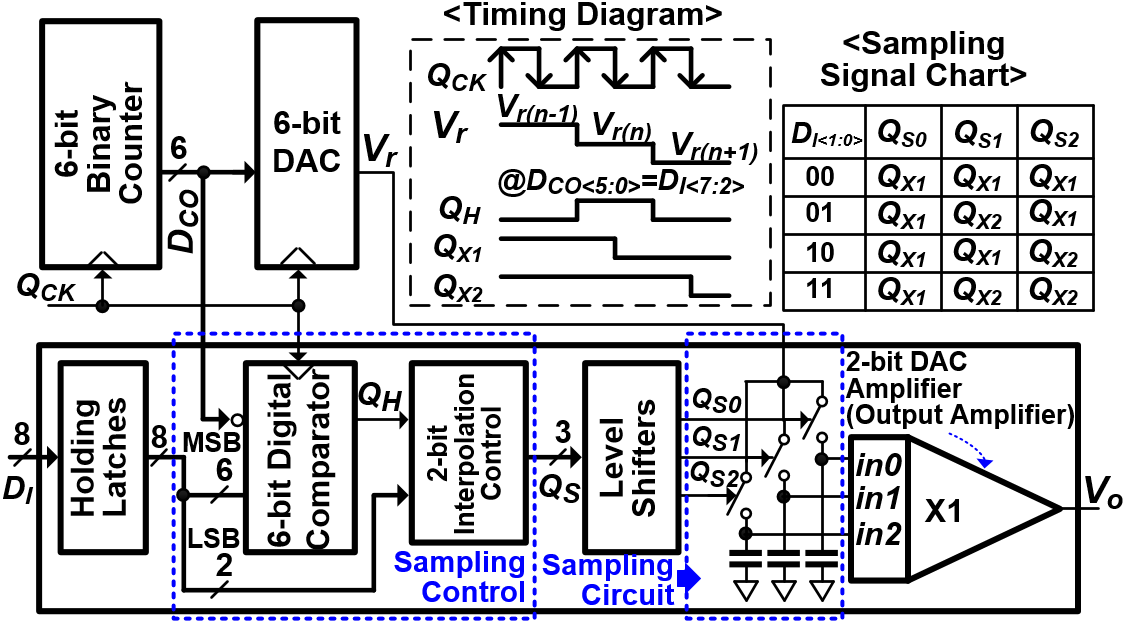Table 2. Inputs and Outputs of the 2-bit DAC Embedded Output Amplifier

 $D_{I<1:0>}$ in0 in1 in2 $V_o$ 00 $V_{r(n)}$ $V_{r(n)}$ $V_{r(n)}$ $V_{r(n)}$ 01 $V_{r(n)}$ $V_{r(n+1)}$ $V_{r(n)}$ (3$V_{r(n)}$+1$V_{r(n+1)}$)/4 10 $V_{r(n)}$ $V_{r(n)}$ $V_{r(n+1)}$ (2$V_{r(n)}$+2$V_{r(n+1)}$)/4 11 $V_{r(n)}$ $V_{r(n+1)}$ $V_{r(n+1)}$ (1$V_{r(n)}$+3$V_{r(n+1)}$)/4

The circuit diagram of the output amplifieris shown in Fig. 7, where the 2 LSB’s interpolation function is added. The employed output amplifier has a class-AB output stage and divides the three input nodes to generate the output voltages between the sampled $V_{r(n)}$ and $V_{r(n+1)}$ depending on 2 LSBs [13-16].

#### 2. Design Considerations of the Ramp-signal Buffer

Fig. 8. Ramp-Signal Buffer Design for the 960-Channel Column Driver IC (a) RC Parasitic of the Ramp-Signal Buffer, (b) the Required Settling Accuracy Estimation.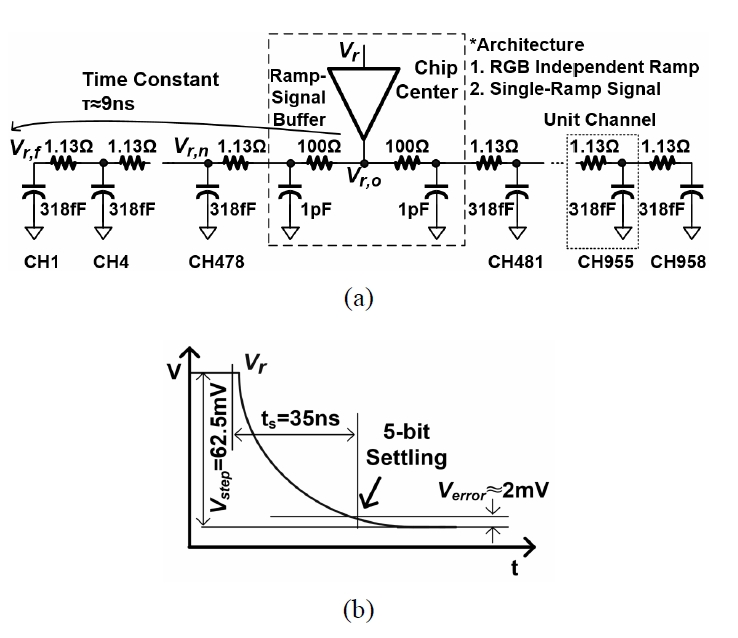Multi-channel column driver IC’s based on the RDAC commonly have a large width of over 10 mm. The global reference voltage circuit of the RDAC tends to be located at the chip center. At this time, the global reference voltage circuit needs to deliver the reference voltage uniformly as far as the outermost channel within the limited 1–H time. Meanwhile, the 1-H time of the 60 Hz/frame UHD panel is 10 μs. If a 2:1 DEMUX function is used, the effective 1-H time of the unit column driver IC should be 3.5 μs . Assuminga settling margin of the output amplifier is 1 μs, the ramp signal of the proposed column driving technique is processed within the time period as given by dividing 2.5 μs by 64 periods. As a result, the unit period of the ramp signal is approximately 40 ns. When the ramp-signal buffer is located at the chip center, its parasitic resistance and capacitance in the 960-channel column driver IC are modeledas Fig. 8(a).

Table 3. Simulated AC Performance of the Ramp-Signal Buffer

 SS, 100 °C TT, 25 °C TT, -40 °C DC Gain 95 dB 104 dB 106 dB Bandwidth 20.9 MHz 28.4 MHz 36 MHz Phase Margin 61.9° 65.9° 69.3° Settling Time 37.3 ns 36.3 ns 35.4 ns RMS Current 1.13 mA 1.17 mA 1.24 mA

In this work, including all parasitic elements, such as routing metals, device parasitics, and sampling capacitors, the parasitic resistance and capacitance of each channel are estimated as 1.13 Ω and 318 fF, respectively, when each channel uses three sampling capacitors of 100 fF. Considering the distance from the ramp-signal buffer to the channels located at the chip center (CH478 and CH481), the additional parasitic resistance and capacitance of 100 Ω and 1 pF, respectively, are also added. At this time, the time constant from the ramp-signal buffer to the outermost channel (CH1) is approximately 9 ns. When the output voltage range is 4 V, the one-step voltage ($V_{step}$) of the 6-bit ramp signal is 62.5 mV as shown in Fig. 8(b). If the voltage accuracy required to the final output of the column driver IC is ±2 mV, the ramp signal delivered to the outermost channel has to be settled to 3.2 % of the target voltage, which is approximately 5-bit resolution, within 40 ns. Under this condition, the required small signal bandwidth ($f_{-3dB}$) of the ramp-signal buffer calculated with Eq. (1)is approximately 18.4 MHz. In Eq. (1), $n$ is the required resolution of the output and ts is the settling time. The width of the routing metal needs to be determined such that 4τ of the RC time constant of the ramp signal from the chip center to outermost channels is within 40 ns.

##### (1)
$f_{- 3dB}=\frac{n\times \ln 2}{2\pi \times ts}$

The designed AC performance of the ramp-signal buffer is summarized in Table 3. As a result of this design, when the required bandwidth is specified under the slowest operation condition (SS, 100 °C), the current consumption of the ramp-signal buffer is 1.13 mA. In the case of the 960-channel column driver IC using the RDAC structure, the current consumption of the global reference voltage circuit is approximately 4 mA. In the proposed column driving technique, taking into account all RGB ramp-signal buffers, the total current consumption is approximately 3.39 mA, which is smaller than the conventional RDAC- based column driver IC.

The simulated waveforms of the ramp signal are shown in Fig. 9. The settling time of the ramp signal at the outermost channel ($V_r$, $f$), CH1, is approximately 37.9 ns under the slowest operation condition.

Fig. 9. Simulated Waveform of the Ramp Signals with the 960-Channel RC Parasitic (a) Settling Behavior, (b) Channel Variations.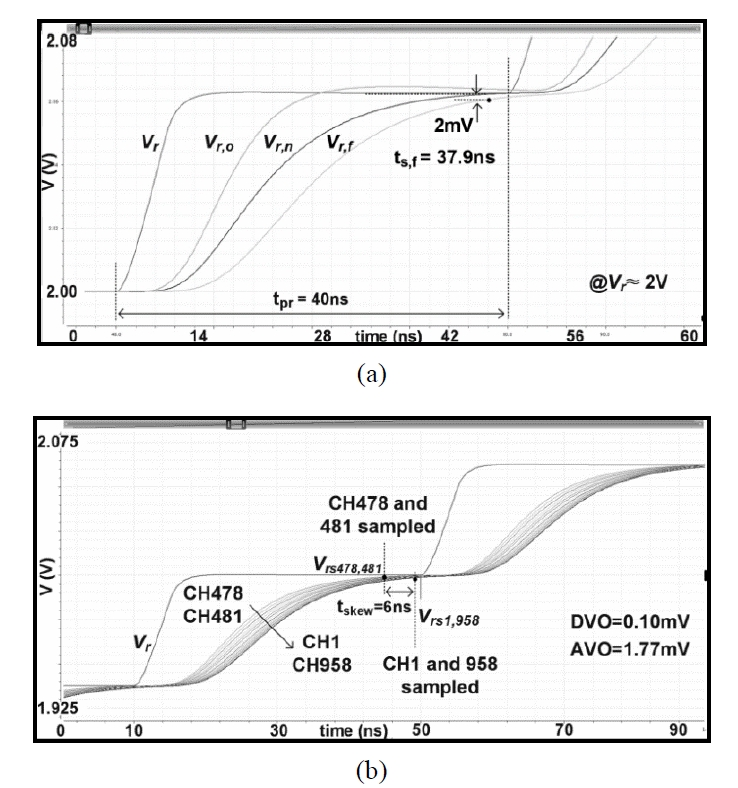Table 4. Simulated DVO and AVO in the 960-Channel Environment

 SS, 100 °C TT, 25 °C TT, -40 °C DVO 0.10 mV 0.32 mV 0.40 mV AVO 1.77 mV 1.52 mV 1.37 mV

Meanwhile, $Q_{CK}$ supplied from the chip center has an RC delay similar to that of the ramp signal. The holding signal ($Q_S$) is generated fastest for CH478 located at the chip center and slowest for CH1, the outermost channel. Froma result of the delay difference of the two extreme signals, the deviation of voltage output (DVO: deviation of voltage output)among the channels within a chipcan be obtained. Since the ramp signal of the outermost channel arrives last, using the absolute-value error at the time, the output voltage amplitude deviation (AVO: output voltage amplitude deviation) can be also obtained. The DVO and AVO of the channels with respect to the operation condition and ramp signal delay are summarized in Table 4. Even under the worst condition, the simulated DVO and AVO are both less than 2 mV, where a target spec is under 8 mV.

#### 3. Sampling Error Reduction for the Ramp Signal-based Column Driving Circuit

In analog voltage sampling circuits, to minimize errors caused by environmental noise, differential-ended signals are mainly used. However, in the AMOLED column driver IC, which should process analog outputs with gamma characteristics, it is not appropriate to use differential-ended signals. Therefore, in this work, a sampling circuit using single-ended signals, which have a small area and simple circuit design, is applied. At this time, the possible errors, which may occur in the ramp signal sampling circuit, are minimized using the analog circuit design techniques as shown in Fig. 10.

Fig. 10. Proposed sampling circuit used to reduce sampling errors.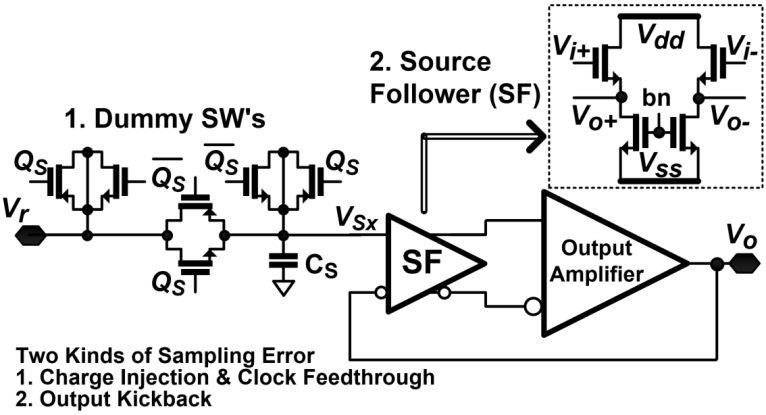If the sampling switch is implemented using a MOS transistor, channel charges can be injected into the source and drain nodes when the MOS transistor turns off. The injected channel charge, $Q_{CH}$, expressed as Formulas (2)and (3)may degrade the image quality of the AMOLED panel. In (2)and (3), $V_{GS}$ is determined as a voltage difference between the sampling signals, $Q_S$, and the input voltage, $V_r$. To minimize sampling errors caused by channel charge injection, a differential-ended signal sampling circuit using the ‘early clock’ and ‘bottom-plate sampling’ is commonly used. However, in the case of the column driver IC, a single-ended signal is used, so it is not easy to apply these circuit design techniques. Furthermore, clock feedthrough generated by the sampling signal and the parasitic capacitance of the sampling switch may degrade DVO and AVO performances. Therefore, in this paper, the CMOS sampling switch and the dummy switches are used simultaneously to minimize channel charge injection and sampling errors caused by clock feedthrough in the rail-to-rail signal range.

##### (2)
$Q_{CH}=W\cdot L\cdot C_{OX}\cdot (V_{GS}- V_{TH}) \\$

##### (3)
$\Delta V_{sx}=\frac{W\cdot L\cdot C_{OX}\cdot (V_{GS}- V_{TH})}{2C_{S}}$

Fig. 11. 20-Channel Layout of the Proposed Column Driver IC.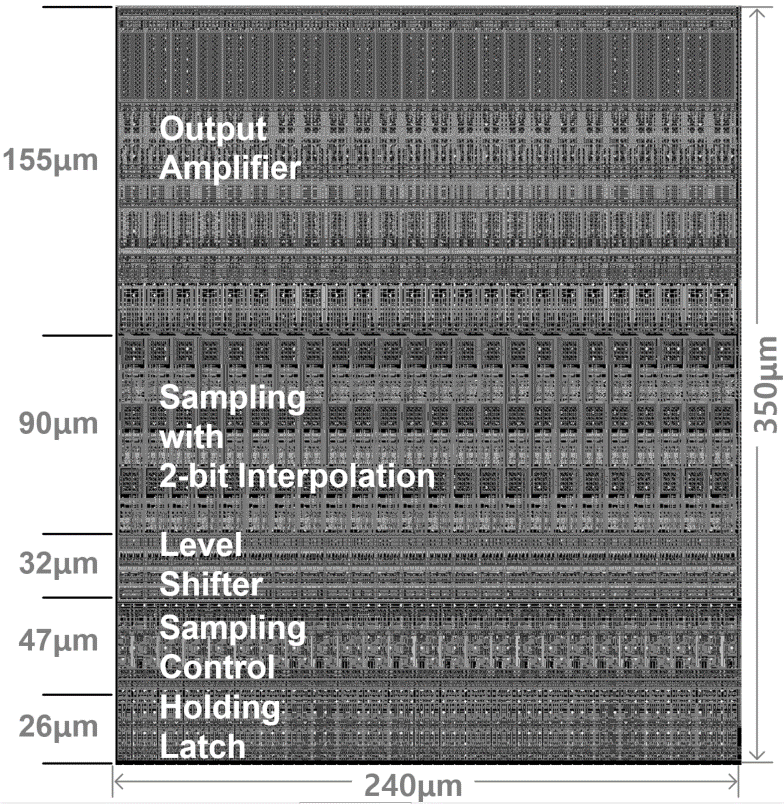The output ($V_o$) connected to the differential-ended input of the output amplifier and the ramp signal ($V_r$) have different settling behaviors from each other. Thus, even after $V_{SX}$ is held at the (+) node of the amplifier, $V_o$ which is connected to the (-) node continues moving towards the target voltage. At this time, output kickback may occur, causing $V_o$ to move the sampled input with $V_{SX}$ . In this paper, errors caused by output kickback are minimized by using the source follower as the input stage of the output amplifier .

### IV. PROTOTYPE MEASUREMENT RESULTS

The 96-channel prototype IC, in which the proposed ramp signal-based column driving technique is applied, is implemented using a 0.18 μm CMOS process. The 20-channel layout is shown in Fig. 11. The effective area per unit channel is 4200 $μm^2$ and when the analog supply voltage and digital supply voltage are 3.3 V and 1.8 V, respectively, the static current consumption per unit channel is 3.0 μA.

The measured output waveforms of a single channel with respect to display pattern are shown Fig. 12. The measured H-stripe pattern waveform is shown in Fig. 12(a) where the output of the unit channel changes rapidly at every 1-H period, while the output amplifier demonstrates the required settling performance of 3.5 μs at worst. The measured H-bar pattern waveform is shown in Fig. 12(b) where the output of the channel varies by 1 LSB duringa single 1-H period, while the gamma output characteristics maintain monotonicity.

Table 5. Performance Comparison with the Previously Reported Column Driver IC’s

  TCASI2010  JSSC2012  JSSC2014  JSSC2013  TCASI2018 This work Resolution [bits] 8 10 10 9 8 8 DAC Type 8b RDAC 6b RDAC + 4b RDAC 7b RDAC + 3b CDAC 6b RDAC + 3b Amp. DAC 6b RDAC + 2b Amp. DAC 6b Ramp + 2b Amp. DAC Analog Supply [V] 5.0 5.0 5.0 5.0 8.0 3.3 Output Range [V] 4.5 4.5 4.5 4.5 5.0 2.4 Max. DVO [mV] 13.2 22.0 5.6 15.9 8.0 6.0 Area/1column [$μm^2$] 40,960 (1280ⅹ32) 13,860 (495ⅹ28) * 5,010 (334ⅹ15) 7,800 (390ⅹ20) * 5,520 (460ⅹ12) 4,200 (350ⅹ12) Process [CMOS] 0.35 μm 0.35 μm 2 Poly 4 Metal 0.11 μm 1 Poly 6 Metal 0.35 μm 2 Poly 4 Metal 0.13 μm 1 Poly 4 Metal 0.18 μm 1 Poly 6 Metal

* The area of the holding latch and level shifters is not included.

Fig. 12. Measured Channel Output Waveform (a) H-Striped, (b) H-Bar Patterns.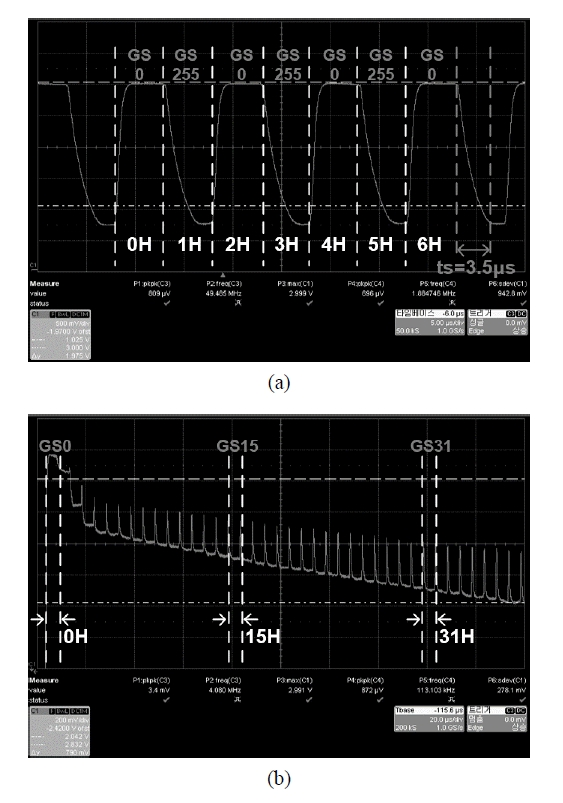The output voltage deviations of the 96-channels are shown in Fig. 13, where the same digital inputs are applied to all channels the 96-channel prototype IC. The measured DVO is within ±6 mV less than a target spec, and from the fact that no particular pattern is found from the chip center to the outermost channel, it is verified that the RC delay of the ramp signal is minimized at the required accuracy.

Fig. 13. Measured DVO Performance of the 96-Channel Prototype IC.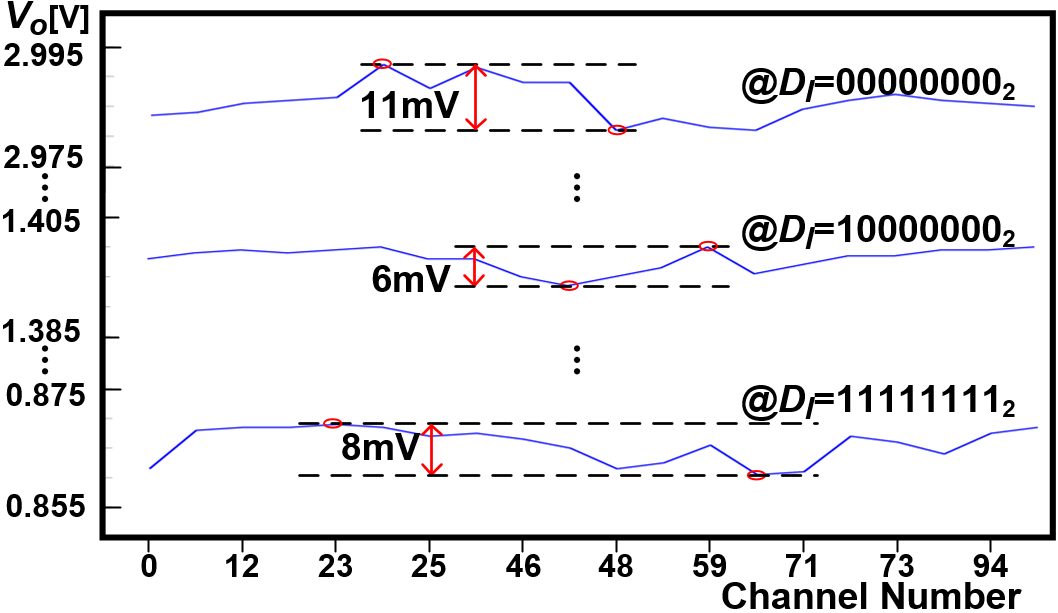The 96-channel prototype IC and the previously presented column driver IC are compared in Table 5. In the proposed ramp signal-based column driver IC, the effective area of the unit channel is 4200 $μm^2$ using the RGB independent ramp signals and 8-bit resolution, which is comparable to the results of other studies. The uniformity based on the DVO is also superior to that of other studies. This indicates that the proposed ramp signal-based column driving technique can effectively minimize the driver IC area while maintaining performance quality.

### V. CONCLUSIONS

This work proposes an area-efficient ramp signal-based column driving technique for high-resolution AMOLED panels. The proposed column driving technique achieves column driver IC area reduction of 44.3% by replacing the conventional RDAC-based column driver IC with a single ramp signal line, while sharing the single ramp signal to vary consecutively in time among all channels. In addition, each unit channel of the proposed column driver IC employs a weighted two-step interpolation topology, composed of the first-stage 6-bit ramp signal and the second-stage 2-bit amplifier DAC, to minimize operation speed, area, and power consumption.

### ACKNOWLEDGMENTS

This work was supported by Samsung Display, the IDEC of KAIST, the Ministry of Trade, Industry & Energy and Korea Semiconductor Research Consortium program for the development of future semiconductor devices (#10080488), and the MSIT under the ITRC program (IITP-2019-2018-0-01421).

### REFERENCES

1
Yoneda K., june. 2001, Development trends of LTPS TFT LCDs for mobile applications, 2001 Symposium on VLSI Circuits. Digest of Technical Papers, 14-16, pp. 85-902
Kim K. M., May 2008, One-Chip Driver IC for 16 Million Color WXGA LTPS TFT LCD Panel, SID Symposium Dig. Tech. Papers3
Lu C., Oct. 2010, An Area-Efficient Fully R-DAC-Based TFT-LCD Column Driver, Circuits and Systems I: Regular Papers, IEEE Transactions on, Vol. 57, No. 10, pp. 2588-26014
Post H.-U., Schoppe K., June 1983, A 14-bit monotonic NMOS D/A converter, Solid-State Circuits IEEE Journal of, Vol. 18, No. 3, pp. 297-3015
Lu C., Oct 2012, A 10-bit Resistor-Floating-Resistor-String DAC (RFR-DAC) for High Color-Depth LCD Driver ICs, Solid-State Circuits IEEE Journal of, Vol. 47, No. 10, pp. 2454-24666
Sung Y. C., April 2006, 10bit Source Driver with Resistor- Resistor-String Digital to Analog Converter, SID Symp. Dig. Tech. Papers7
Umeda K., May 2008, A novel linear digital-to-analog converter using capacitor coupled adder for LCD driver ICs, SID Symp. Dig. Tech. Papers8
Kim H. S., March2014, A 10-Bit Column-Driver IC With Parasitic-Insensitive Iterative Charge-Sharing Based Capacitor-String Interpolation for Mobile Active-Matrix LCDs, Solid-State Circuits, IEEE Journal of, Vol. 49, No. 3, pp. 766-7829
Jeon Y. J., Dec 2009, A Piecewise Linear 10 Bit DAC Architecture With Drain Current Modulation for Compact LCD Driver ICs, Solid-State Circuits, IEEE Journal of, Vol. 44, No. 12, pp. 3659-367510
Lu C., June 2013, A 10-b Two-Stage DAC with an Area-Efficient Multiple-Output Voltage Selector and a Linearity-Enhanced DAC-Embedded Op-Amp for LCD Column Driver ICs, Solid-State Circuits, IEEE Journal of, Vol. 48, No. 6, pp. 1475-148611
Lu C., Oct 2014, A Column Driver Architecture With Double Time-Division Multiplexing RDACs for TFT-LCD Applications, Solid-State Circuits, IEEE Journal of, Vol. 49, No. 10, pp. 2352-236412
Li H. G., May 2015, High-Precision Mixed Modulation DAC for an 8-Bit AMOLED Driver IC, Display Technology, IEEE Journal of, Vol. 11, No. 5, pp. 423-42913
Kang J. S., Dec 2007, 10-bit Driver IC Using 3-bit DAC Embedded Operational Amplifier for Spatial Optical Modulators (SOMs), Solid-State Circuits, IEEE Journal of, Vol. 42, No. 12, pp. 2913-292214
Hogervorst R., Dec 1994, A compact power-efficient 3 V CMOS rail-to-rail input/output operational amplifier for VLSI cell libraries, Solid-State Circuits, IEEE Journal of, Vol. 29, No. 12, pp. 1505-151315
Kim Y. J., March 2010, A 12 bit 50 MS/s CMOS Nyquist A/D Converter With a Fully Differential Class-AB Switched Op-Amp, Solid-State Circuits, IEEE Journal of, Vol. 45, No. 3, pp. 620-62816
An et al. T. J., Oct 2018, Area-Efficient Time-Shared Digital-to-Analog Converter With Dual Sampling for AMOLED Column Driver IC’s, Circuits and Systems I: Regular Papers, IEEE Transactions on, Vol. 65, No. 10, pp. 3227-324017
Park Y. S., Feb 2007, An 8b Source Driver for 2.0 inch Full-Color Active-Matrix OLEDs Made with LTPS TFTs, 2007 IEEE International Solid-State Circuits Conference. Digest of Technical Papers, 11-15, pp. 130-592## Author

Tai-Ji An received the B.S. degree in electronic engineering from University of Seoul, Korea, in 2007, and the M.S. and Ph.D. degrees in electronic engineering from Sogang University, Korea, in 2013 and 2019, respectively.

From 2007 to 2011, he was with Luxen Technologies, where he had developed various power-management and analog integrated circuits.

Dr. An has been with the Samsung Electronics Co., Ltd. and has developed power monitoring IP’s for low-power mobile application processors.

Moon-Sang Hwang received the B.S., M.S. and Ph.D degrees in electrical engineering from Seoul National University, Korea, in 2000, 2003 and 2009, respectively.

Since 2009, Dr. Hwang has been with the Samsung Display Co., Ltd. and has developed display driver IC’s and high-speed interface for display driver IC and timing controller.

His current research interests include design and developing of low-power and area efficient display driver IC architecture, high-speed interface for display driver IC, small-size data converters, sensors and mixed-mode integrated system.

Won-Jun Choe was born in Busan, 1969. 2. 9. He've got Ph.D., M.S. and B.S. degrees from E.E. of Seoul National University, E.E. of Seoul National University, and E.E. of Pusan National University respec-tively.

He has worked for Samsung Display since 2012, and he has 25 years experience on display electronics. His major is about circuitary for display driving, and display interface technologies.

Recently, he has researched OLED driving technology, especially about uniformity, life time, and high BW interface.

Jun-Sang Park received the B.S. and M.S. degrees in electronic engineering from Sogang University, Seoul, Korea, in 2012 and 2014, respectively, where he is currently pursuing the Ph.D. degree.

He is a recipient of a scholarship sponsored by Samsung electronics.

His current interests are in the design of high-resolution low-power CMOS data converters, PMICs, and very high-speed mixed-mode integrated systems.

Gil-Cho Ahn received the B.S. and M.S. degrees in electronic engi-neering from Sogang University, Seoul, Korea, in 1994 and 1996, respectively, and the Ph.D. degree in electrical engineering from Oregon State University, Corvallis, in 2005.

From 1996 to 2001, he was a Design Engineer at Samsung Electronics, Kiheung, Korea, working on mixed analog-digital integrated circuits. From 2005 to 2008, he was with Broadcom Corporation, Irvine, CA, working on AFE for digital TV.

Currently, he is a Professor in the Department of Electronic Engineering, Sogang University.

His research interests include high-speed, high-resolution data converters and low-voltage, low-power mixed-signal circuits design.

Seung-Hoon Lee received the B.S. and M.S. degrees in electronic engineering from Seoul National University, Korea, in 1984 and 1986, respectively, and the Ph.D. degree in electrical and computer engineering from the University of Illinois, Urbana-Champaign, in 1991.

He was with Analog Devices Semiconductor, Wilmington, MA, from 1990 to 1993, as a Senior Design Engineer.

Since 1993, he has been with the Department of Electronic Engineering, Sogang University, Seoul, where he is currently a Professor.

His current research interests include design and testing of high-resolution high-speed CMOS data converters, CMOS communication circuits, integrated sensors, and mixed-mode integrated systems.

Dr. Lee has been a member of the editorial board and the technical program committee of many international and domestic journals and conferences including the IEEK Journal of Semiconductor Devices, Circuits, and Systems, the IEICE Transactions on Electronics, and the IEEE Symposium on VLSI Circuits.